## Tag: Keras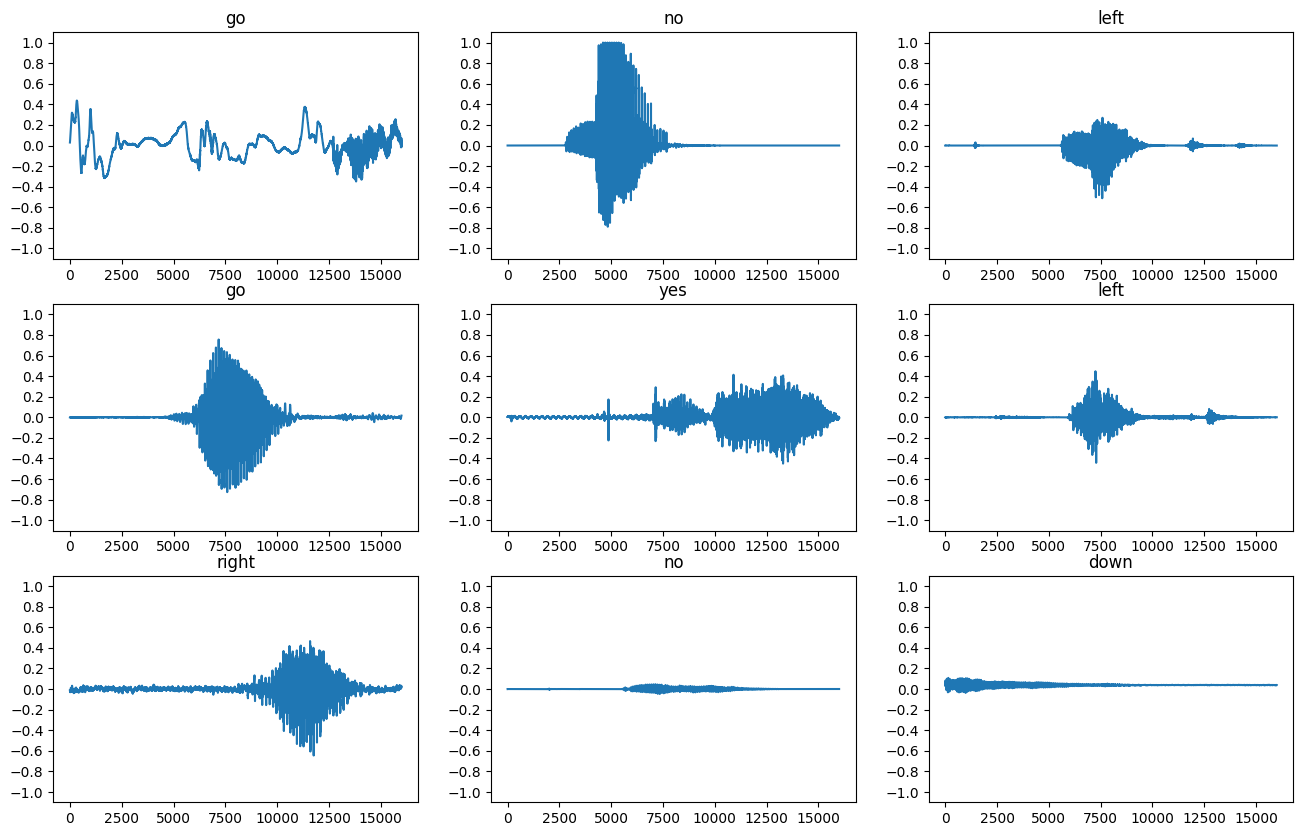### Simple audio recognition: Recognizing keywords

Simple audio recognition: Recognizing keywords Simple audio recognition: Recognizing keywords  |  TensorFlow Core "This tutorial will show you how to build a basic speech recognition network that recognizes ten different words. It's important t …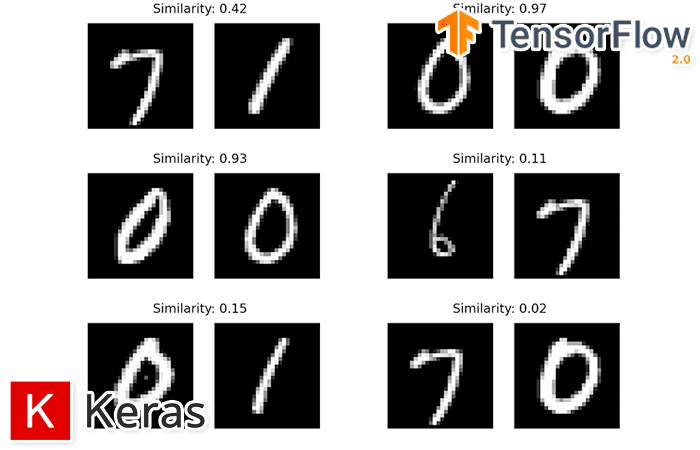### Comparing images for similarity using siamese networks, Keras, and TensorFlow

Comparing images for similarity using siamese networks, Keras, and TensorFlow Comparing images for similarity using siamese networks, Keras, and TensorFlow - PyImageSearch In this tutorial, you will learn how to compare two images for similarit …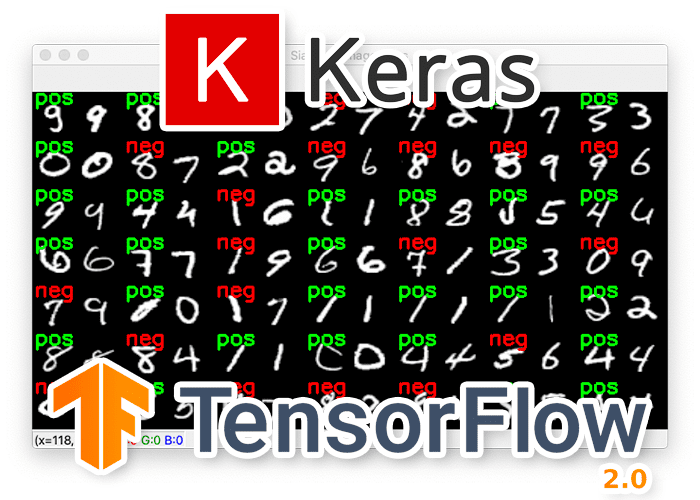### Building image pairs for siamese networks with Python

Building image pairs for siamese networks with Python Building image pairs for siamese networks with Python - PyImageSearch In this tutorial you will learn how to build image pairs for training siamese networks. We’ll implement our image pair g …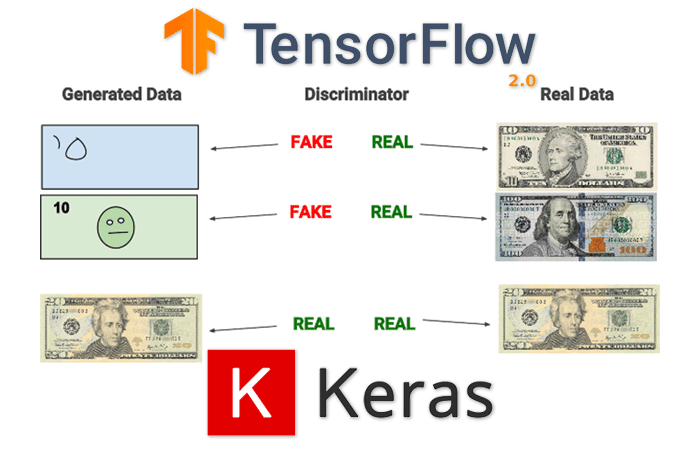### GANs with Keras and TensorFlow

GANs with Keras and TensorFlow GANs with Keras and TensorFlow - PyImageSearch In this tutorial you will learn how to implement Generative Adversarial Networks (GANs) using Keras and TensorFlow. Source: www.pyimagesearch.com/2020/11/16/gans-wit …### AI Queue Length Detection: Counting the Number of People in an Area

AI Queue Length Detection: Counting the Number of People in an Area AI Queue Length Detection: Counting the Number of People in an Area In this article, we will train a deep learning model to detect and count the number of people in a given are …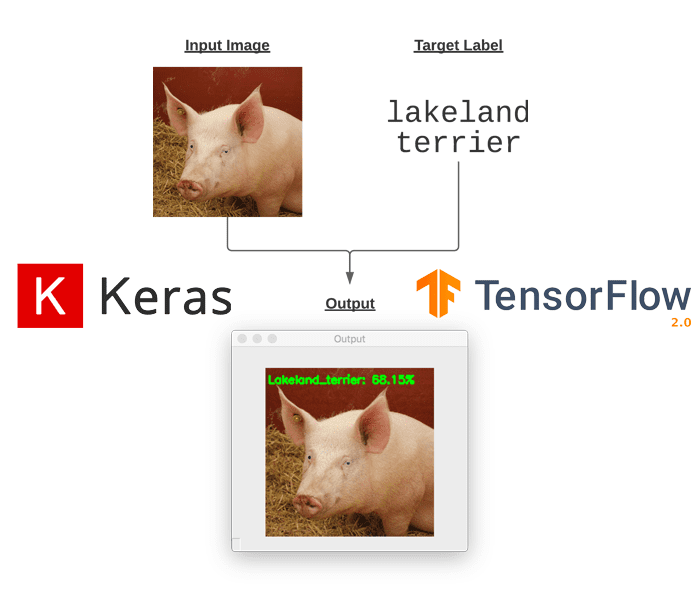### Targeted adversarial attacks with Keras and TensorFlow – PyImageSearch

Targeted adversarial attacks with Keras and TensorFlow Targeted adversarial attacks with Keras and TensorFlow - PyImageSearch In this tutorial, you will learn how to perform targeted adversarial attacks and construct targeted adversarial images …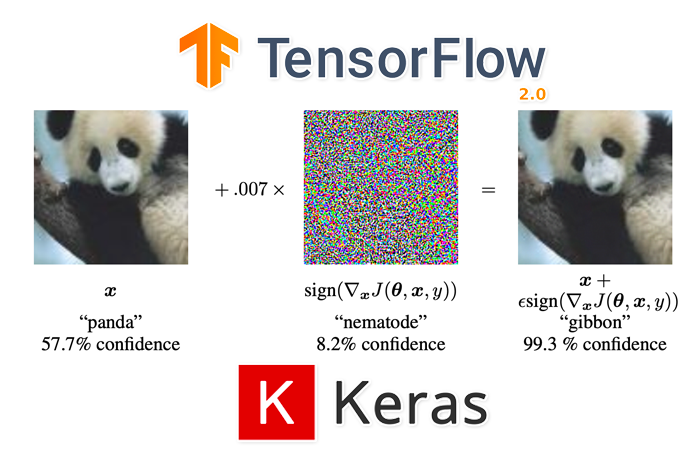### Adversarial images and attacks with Keras and TensorFlow

Adversarial images and attacks with Keras and TensorFlow Adversarial images and attacks with Keras and TensorFlow - PyImageSearch In this tutorial, you will learn how to break deep learning models using image-based adversarial attacks. We will …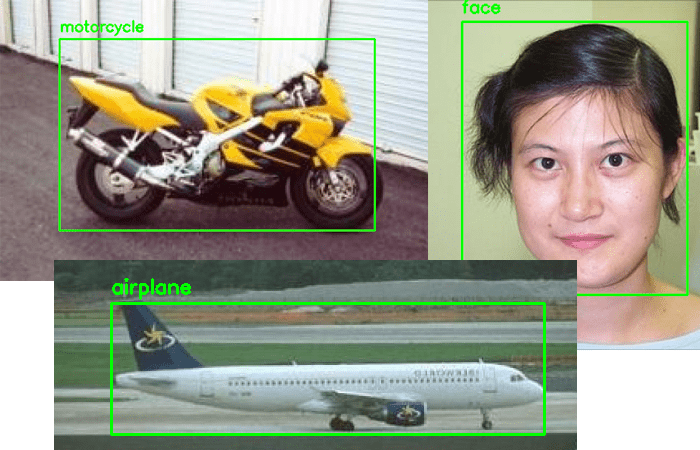### Multi-class object detection and bounding box regression with Keras, TensorFlow, and Deep Learning

Multi-class object detection and bounding box regression with Keras, TensorFlow, and Deep Learning Multi-class object detection and bounding box regression with Keras, TensorFlow, and Deep Learning - PyImageSearch In this tutorial, you will lea …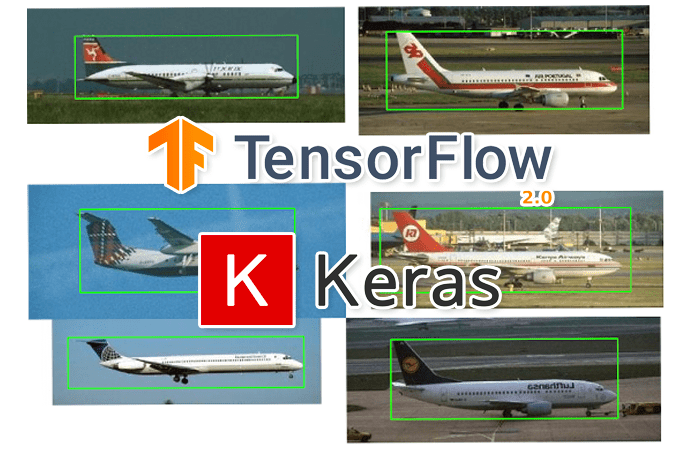### Object detection: Bounding box regression with Keras, TensorFlow, and Deep Learning

Object detection: Bounding box regression with Keras, TensorFlow, and Deep Learning Object detection: Bounding box regression with Keras, TensorFlow, and Deep Learning - PyImageSearch In this tutorial you will learn how to train a custom deep l …### An Introduction to Adversarial Examples in Deep Learning

An Introduction to Adversarial Examples in Deep Learning Weights & Biases This report provides an intuitive introduction to adversarial examples, discusses a wide variety of different adversarial attacks and, most notably, provides advice o …

Subscribe to our Digest